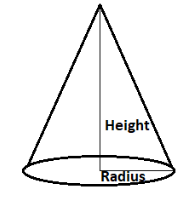QUESTION

# If the area of the base of a right circular cone is 51${m^2}$ and volume is 68${m^3}$ then its vertical height is A. 3.5 mB. 4 mC. 4.5 mD. 5 m

Hint:- Let us find the radius of the right circular cone from the given area of the base of the cone. After that we will get the height of the cone by putting the value of radius in the formula of volume of cone.As we know that the base of the right circular cone is of the shape of the circle.
And the formula for the area of the circle is $\pi {r^2}$(where r is the radius of the circle).
So, as we know that the area of the base is 51${m^2}$.
So, let the radius of the cone is r metres.
So, $\pi {r^2}$ = 51
So, dividing both sides of the above equation by $\pi$. We get,
${r^2} = \dfrac{{51}}{\pi }$
$r = \sqrt {\dfrac{{51}}{\pi }}$ metres.
Now as we know that the formula for calculating the volume of the right circular cone is $\dfrac{1}{3}\pi {r^2}h$ (where r is the radius and h is the height of the cone)
Let the height of the right circular cone is h metres.
So, now we know the volume and radius of the cone. So, we can easily find the height of the cone by putting the value of radius in the formula of volume of cone.
So, $\dfrac{1}{3}\pi {r^2}h$ = 68 (1)
Now to find the value of h we had to put the value of r in the above equation. We get,
$\dfrac{1}{3}\pi {\left( {\sqrt {\dfrac{{51}}{\pi }} } \right)^2}h$ = 68
$\dfrac{{51}}{3}h$ = 68
Now multiplying both sides of the above equation by $\dfrac{3}{{51}}$. We get,
h = $\dfrac{{68 \times 3}}{{51}}$ = $\dfrac{{12}}{3}$ = 4 metres.
So, the height of the given right circular cone will be 4 metres.
Hence, the correct option will be B.

Note:- Whenever we come up with this type of question where we are given the volume of cone and asked to find the height of it then we first write the formula for volume of cone i.e. $\dfrac{1}{3}\pi {r^2}h$. And then check which of the parameters other than height are not given to us(like here radius). And then we find the value of radius using by equating the given area with the formula for area of circle. After that we put the radius in the volume equation and easily find the height of the cone.